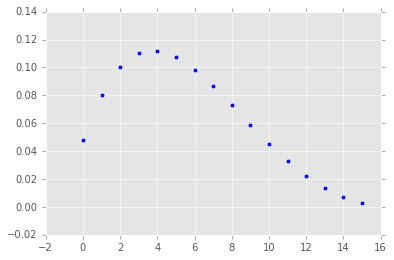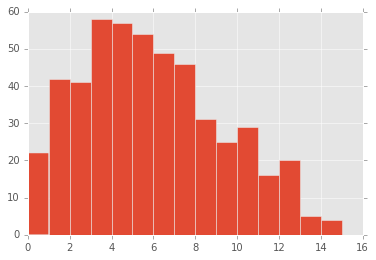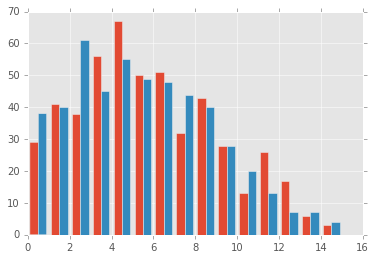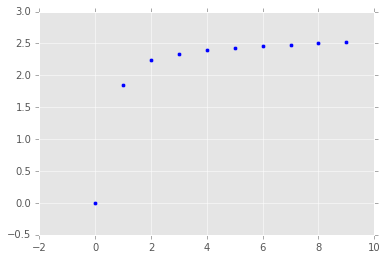# Explaining 'Explaining the Gibbs Sampler'¶

Eamonn Bell, Columbia University [email protected]

Example taken from Casella, George, and Edward I. George. "Explaining the Gibbs Sampler." The American Statistician 46, no. 3 (1992): 167-74.

https://www.jstor.org/stable/2685208

In :
import scipy as sp

import numpy as np

from matplotlib import pyplot as plt
from scipy.special import comb, betaln

In :
%matplotlib inline
plt.style.use('ggplot')


Consider a joint of $X, Y$:

$$f(x,y) \propto \binom{n}{x} y^{x + \alpha - 1}(1 - y)^{n - x + \beta -1 }$$

We can get the marginal $f(x)$ analytically (the beta-binomial)

$$f(x) = \binom{n}{x} \frac{\Gamma \left( \alpha + \beta \right)}{\Gamma \left( \alpha \right)\Gamma \left( \beta \right)} \frac{\Gamma \left( x + \alpha \right)\Gamma \left( n - x + \beta \right)}{\Gamma \left( \alpha + \beta + n \right)}$$
In :
# http://www.channelgrubb.com/blog/2015/2/27/beta-binomial-in-python

def BetaBinomAnalytic(alpha,beta,n,x):
# log sum exp
part_1 = comb(n,x)
part_2 = betaln(x+alpha,n-x+beta)
part_3 = betaln(alpha,beta)

result = (np.log(part_1) + part_2) - part_3

return np.exp(result)


Let

In :
ALPHA = 2
BETA = 4
N = 16

In :
x = np.arange(0, 16, 1)
y = [BetaBinomAnalytic(alpha=ALPHA, beta=BETA, n=N, x=x_i) for x_i in x]

In :
plt.scatter(x, y)

Out:
<matplotlib.collections.PathCollection at 0x7f39a3a171d0>We might also want to use scipy rvs interface to construct something that will give us samples

In :
def BetaBinomialSampler(alpha, beta, n, size):

# draw size samples from the beta
beta_samples = sp.stats.beta.rvs(a=alpha,b=beta,size=size)

beta_binomial_samples = []

for p_from_beta in beta_samples:
sample = sp.stats.binom(n=n, p=p_from_beta).rvs()
beta_binomial_samples.append(sample)

return np.array(beta_binomial_samples)

In :
plt.hist(BetaBinomialSampler(alpha=ALPHA, beta=BETA, n=N, size=500),bins=np.arange(16))
plt.show()In this case, it is possible to get the marginal analytically ($p(x) = \frac{p(x,y)}{p(y \mid x)}$). We had to do that to compute the first function above.

The idea behind Gibbs sampling is to get draws from a distribution that is "similar enough" to this marginal, so that when it's hard to compute the distribution analytically we aren't stuck.

### Gibbs sequence¶

To do this, we consider a "Gibbs sequence" of random variables that are related to the conditional distributions $f(x \mid y)$ and $f(y \mid x)$ in a special way.

$$Y'_0, X'_0, Y'_1, X'_1, \ldots, Y'_k, X'_k$$

Where some initial value $Y'_0 = y'_0$ is specified, and the following relations hold:

$$X'_j \sim f(x \mid Y'_j = y'_j)\\ Y'_{j + 1} \sim f(y \mid X'_j = x'_j)$$

The idea is that it is generally easier to specify these conditionals analytically than the marginal.

It turns out that as $k \rightarrow \infty$, $X'_k = x'_k$ is effectively a sample from $f(x)$. This is what the Gibbs sampler does.

### Applied¶

In the present case: $f(x \mid y)$ is $\textrm{Binomial}(n, y)$, while $f(y \mid x)$ is $\textrm{Beta}(x + \alpha, n - x + \beta)$. Lets wrap the scipy rvs interface with our parameters to get some samplers.

In [ ]:
def x_given_y(y):
return sp.stats.binom(n=N, p=y).rvs()

def y_given_x(x):
return sp.stats.beta(x + ALPHA, N - x + BETA).rvs()


The sampler implements the relationships above.

In :
def GibbsSampler(k, initial_state):
y_0 = initial_state

y_current = y_0
x_current = 0

for _ in range(k):
# implements relations above
x_current = x_given_y(y_current)
y_next = y_given_x(x_current)

# update for next iteration
y_current = y_next

return x_current


Now, we generate 500 samples each from the analytic solution (the Beta-Binomial) and the Gibbs-sampled marginal (with k iterations), respectively.

In :
analytic_samples = BetaBinomialSampler(alpha=ALPHA, beta=BETA, n=N, size=500)

gibbs_initial = 0.01
gibbs_samples = [GibbsSampler(10, initial_state=gibbs_initial) for _ in range(500)]

In :
plt.hist([analytic_samples, gibbs_samples], bins=np.arange(16))
plt.show()It's important to make sure the sampler is settling down on a good estimate of the distribution.

In :
def GibbsSamplerWithHistory(k, initial_state):
y_0 = initial_state

y_current = y_0
x_current = 0

y_history = []
x_history = []

for _ in range(k):
x_history.append(x_current)
y_history.append(y_current)

# implements relations above
x_current = x_given_y(y_current)
y_next = y_given_x(x_current)

# update for next iteration
y_current = y_next

return x_history, y_history


Make a bunch of draws, and study the behavior of the sampler by looking at the entropy of the distribution generated if we stopped the sampler in its tracks at the kth iteration.

In :
MAX_K = 10

histories = []

for _ in range(500):
x_history, y_history = GibbsSamplerWithHistory(k=MAX_K, initial_state=0.187)
histories.append((x_history, y_history))

In :
def dist_at_iteration(k):
dist = []
for history in histories:
dist.append(history[k])
return dist

In :
def normalize(d):
raw = sum(d.values())
return {key:value/raw for key,value in d.items()}

In :
from collections import Counter

ks = []
entropies = []

for k in range(MAX_K):
c = Counter(dist_at_iteration(k))
H = sp.stats.entropy(list(normalize(c).values()))
entropies.append(H)
ks.append(k)

In :
plt.scatter(ks, entropies)
plt.show()